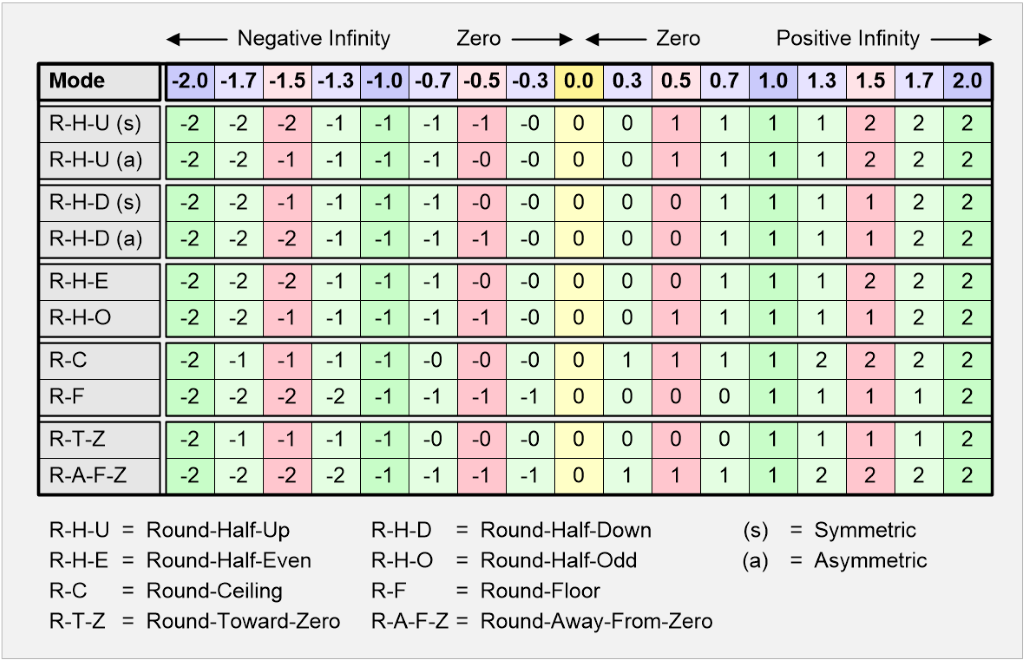# Rounding Numbers Calculator

Created by Mateusz Mucha and Maciej Kowalski, PhD candidate
Reviewed by Jack Bowater
Last updated: Apr 06, 2022

This rounding numbers calculator can be used to decrease the precision of a number to make it shorter, simpler and/or easier to grasp when you perform further operations on it. It's often the case you don't need an exact number (such as 3324.238576) and would happily settle for 3324.34, 3324 or even 3300; this rounding decimals calculator will let you do just that. Simply enter a number and pick the desired precision from the list. If this isn't for you, you may pick a rounding mode.

## Rounding modes

Depending on the situation, you will want this rounding algorithm to behave appropriately. Sometimes you want to round everything up (2.1 would be rounded to 3). Sometimes you want exact halves to be rounded up in half of the cases and down in the other half, in order to for a higher chance of an average staying close to the truth. Here's the description of all modes, we'll round to the nearest whole number.

• up - rounds away from zero. 3.2 and 3.6 become 4, but -3.2 and -3.6 become -4.
• down - rounds towards zero. The above numbers become 3 and -3 respectively.
• ceil - rounds towards the larger number. It differs from rounding up by the way it handles negative numbers. Both -3.2 and -3.6 become -3.
• floor - it rounds towards the smaller number. Similarly, negative numbers go the opposite way than in the case of rounding down. -3.2 becomes -4. This principle is what our modulo calculator is based on.
• half up (default) - rounds towards the nearest neighbor. If equidistant, it rounds away from zero (just like in the up mode). For example, 3.5 becomes 4 and -3.5 becomes -4. This is how rounding is usually performed.
• half down - similarly to half up, it rounds to the nearest neighbor, unless it's equidistant - then it rounds towards zero (just like in the down mode). The above numbers become 3 and -3 respectively.
• half even - an interesting one. Rounds towards the nearest neighbor, but if equidistant, it rounds towards the even number. Both 1.5 and 2.5 are rounded to 2. 3.5 and 4.5 are rounded to 4. It prevents cumulative rounding errors and this is why it's often used in science (we use it as a default mode in our sig fig calculator. If you'd round up all the time, the average is too high... this is why we round up half the time. The same rule (for the same reason) is used in accounting. When you want to round to the nearest cent, just use the half even mode.
• half ceil - the nearest neighbor, equidistant values go towards the larger number.
• half floor - the nearest neighbor, equidistant towards the smaller number.

## Rounding at a glance

If all this text sounds daunting here is a quick table where you can check and understand all the rounding modes available in the calculator as well as some extras as a bonus. Credit where credit is due: this table has been taken from a by Max Maxfield, a recommended read.Mateusz Mucha and Maciej Kowalski, PhD candidate
Number
Round to
Hundredths (0.01)
Result
For non-standard rounding modes check out the advanced mode.
Example
NumberApproximation
-1.237-1.24
-1.235-1.24
-1.233-1.23
4.5674.57
4.5654.57
4.5634.56
People also viewed…

### Circumference of a cylinder

Use this circumference of a cylinder calculator to find a circumference of a cylinder.

### Factoring trinomials

This factoring trinomials calculator can show you step-by-step how to factor a quadratic trinomial using the method of grouping (a.k.a. the ac method).

### Grams to cups

The grams to cups calculator converts between cups and grams. You can choose between 20 different popular kitchen ingredients or directly type in the product density.

### Meat footprint

Check out the meat impact - on the environment and your health.